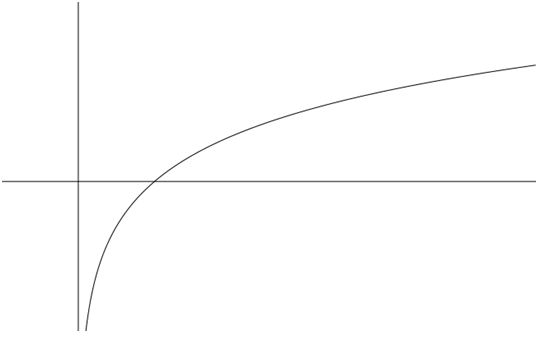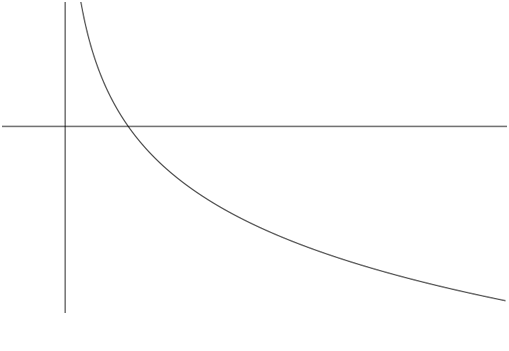# Section 4.3 - Logarithmic Functions

Section Objectives

1. Convert between logarithmic and exponential notation.
2. Evaluate logarithmic functions.
3. Graph logarithmic functions.
4. Use properties of logarithms to simplify expressions.

### Logarithmic Functions

The logarithmic functions are the inverses of the exponential functions.

To more specific...

Let $a$ be a fixed positive real number not equal to 1. The logarithmic function with base-$a$, denoted $\log_a ⁡x$, is the inverse of the base-$a$ exponential function. That is,

#### Examples

• $\log_2 1024 = 10$ because $2^{10} = 1024$.

• $\log_{10} 1000 = 3$ because $10^3=1000$.

• Can you find two consecutive positive integers that bound $\log_3 20$?

• Your calculator should compute base-10 logarithms, often called common logs. Use your calculator to compute $\log_{10} 37=\log 37$.

### Properties of the Logarithmic Functions

Because the logs and exponentials are inverses, we must have:

• $\log_a⁡ (a^x) = x$ for any real number $x$
• $a^{\log_a ⁡x} = x$ for any positive real number $x$

#### Examples

• $\log_5 5^8 = 8$

• $10^{\log 15} = 15$

In general, the logarithmic functions have the following properties.

#### $f(x)=\log_a x, a>1$

• Continuous and increasing
• One-to-one ( Graph passes the horizontal line test.)
• Domain: $(0, +\infty)$, i.e., all positive real numbers
• Range: $(-\infty,+\infty)$, i.e., all real numbers
• $x=0$ is a vertical asymptote of the graph.
• $(1,0)$ is the only $x$-intercept of the graph.
• $(a,1)$ is a point on the graph.
• $f(x) \to \infty$ as $x \to \infty$, but it does so slowly.#### $f(x)=\log_a x, 0

• Continuous and decreasing
• One-to-one ( Graph passes the horizontal line test.)
• Domain: $(0, +\infty)$, i.e., all positive real numbers
• Range: $(-\infty,+\infty)$, i.e., all real numbers
• $x=0$ is a vertical asymptote of the graph.
• $(1,0)$ is the only $x$-intercept of the graph.
• $(a,1)$ is a point on the graph.
• $f(x) \to -\infty$ as $x \to \infty$, but it does so slowly.#### Examples

• Discuss the graph of $y=\log_3 x$.

• Discuss the graph of $y=\log_{2/3} x$.

• Discuss the graph of $y=1+\log_2 (x-4)$.

### The Natural Logarithm

The base-$e$ logarithm is called the natural logarithm:

Your scientific calculator has built-in functions to compute base-10 and base-$e$ exponentials and logarithms.

### Using the Properties of Logs

The properties of logarithms can be very useful when evaluating expressions and solving equations.

#### Examples

• Solve for $x$: $\quad \log(2x+1)=\log 3x$

• Solve for $x$: $\quad \log_4(x^2-6)=\log_4 10$

• Use the properties of logs to evaluate $\log_2 \frac{1}{8}$.

• Use the properties of logs to evaluate $\log_3 \sqrt{9}$.

• Use the properties of logs to evaluate $\displaystyle \ln \frac{1}{e^2}$.